# Demand curve estimation

Demand curve estimation refers to the exercise of estimating the demand curve, typically the market demand curve (as opposed to the individual demand curve) for a good. Demand curve estimation is typically done for the following purposes:

• It may be done by sellers (and in some cases buyers) with significant market power, so that they can decide the appropriate price to set. Note that buyers or sellers who do not have market power simply set the price as the market price and know that whatever quantity they produce will get sold. In contrast, in the extreme case of a monopoly, the seller chooses both the price and quantity but is not guaranteed to sell everything. In order to be so guaranteed, the seller needs to have a plot of the market demand curve so that the (price,quantity) pair can be chosen as a point on the demand curve.
• It may be done by buyers or sellers in order to better estimate prices and quantities to buy or sell for the future. Note that this is advantageous even in a perfectly competitive market: with knowledge of the future demand and supply curves, sellers can estimate future prices, and therefore optimize their long-run choices (i.e., make appropriate fixed cost investments).

## Rules of thumb

• By the law of demand, demand curves tend to be downward-sloping.
• The price-elasticity of demand tends to be higher for luxury goods than for necessity goods.
• The price-elasticity of demand is higher the more specific the category of goods. For instance, the price-elasticity of demand for Pepsi would be greater than the price-elasticity of demand for cold drinks at large.
• Long-run demand tends to be more price-sensitive (i.e., price-elasticity of demand is higher) than short-run demand.

Although these rules of thumb do not directly allow us to construct demand curves empirically, they are helpful when pieced together with other data and also in providing a sanity check.

## The main challenge

Roughly speaking, the following are some reasons for the difficulty of demand curve estimation:

• At any given point in time, one can observe only one point on the demand curve, i.e., one tuple of (price, quantity) values.
• At different points in time, we observe different (price, quantity) values. But it is not clear whether these different (price, quantity) values are on the same demand curve. In other words, it is difficult to separate the role of changes in demand and changes in supply in explaining the different (price, quantity) values.

## The use of regression analysis

The tool of choice for empirical demand curve estimation is regression. The idea is that we write a general functional form with unknown parameters that expresses the quantity demanded as a function of price and other determinants of demand, some of which may differ in value across the different (price,quantity) observations we have. We then combine all the (price,quantity) observations with empirical estimates of the other determinants of demand for each of the instances under consideration. We use the methods of regression (such as least squares regression or logistic regression) to find the values of the unknown parameters and thereby obtain a concrete expression for the demand function. This expresses quantity demanded as a function of price and other determinants of demand. Using estimates of the values of these other determinants of demand in the context where we want the demand curve estimated, we can draw the demand curve.

When individual demand curve data is available, it may be best to perform regression analysis on this data to estimate individual demand curves, then add up across all the individual buyers to obtain the market demand curve. If individual demand curve data is not available, we use the market demand curve in conjunction with aggregated data for the determinants of demand.

For instance, consider the problem of determining the household demand curve for milk. Let us say that we think that the quantity of milk demanded by a household can be (approximately) predicted based on the household income, number of children, total number of family members, and education level of the household members. We write a general functional form (with some unknown parameters) that expresses the quantity of milk purchased in terms of these determinants of demand. We now collect a lot of data on the amount of milk that different households purchased at different prices, along with the values of the various determinants of demand for these households. We then plug these into a regression analysis to estimate the values of the parameters. Note that the regression analysis can be used not just to find the parameters but also to test how good the hypothesis is.

### Logarithmic transformation for a power-based functional form

A typical choice of functional form is where we estimate the quantity demanded to be a constant times a product of powers of each of the determinants of demand, with the exponents used being the unknown parameters and the constant. For instance, if the quantity demanded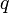$q$ is assumed to be a function of price$p$ and four other quantitative measures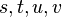$s,t,u,v$, we expect the functional form: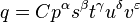$q = Cp^\alpha s^\beta t^\gamma u^\delta v^\varepsilon$

This is not a linear expression, so linear regression cannot be applied directly. In order to apply linear regression, we take logarithms on both sides: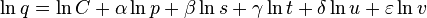$\ln q = \ln C + \alpha \ln p + \beta \ln s + \gamma \ln t + \delta \ln u + \varepsilon \ln v$

We can now apply linear regression to estimate the parameters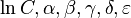$\ln C, \alpha, \beta, \gamma, \delta, \varepsilon$. The typical approach uses ordinary least squares regression. Note that since ordinary least squares regression penalizes based on the square of the additive error after taking logarithms, it is penalizing based on the square of the logarithm of the multiplicative error before taking logarithms. This makes sense if we expect the errors to grow in proportion to the quantities being estimated.

Note also that if we are using a functional form of the above sort, and taking logarithms prior to the regression, it is important that we use demand curve data at the level of granularity that our demand function operates, which is usually the individual level. The problem with market-level data is that the above functional form (product of powers of functions) does not interact well with additive aggregation. Rather, it interacts well with multiplicative aggregation. Thus, if we have access to only market-level data rather than individual-level data, we should use the average values and the distribution to estimate the geometric averages for the quantity demanded and for each of the determinants of demand before plugging into the regression.

### The use of logistic regression

In some cases, the decision at the level of individual buyers boils down to a yes/no decision: do they buy it or do they not? In this case, the above type of model is less useful. There are two alternatives:

• Model the reservation price as a function of other determinants of demand, using linear regression techniques similar to the above.
• Model the decision of whether or not to buy as a probabilistic decision obtained by applying the logistic function to an expression of the above logarithmic form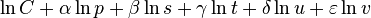$\ln C + \alpha \ln p + \beta \ln s + \gamma \ln t + \delta \ln u + \varepsilon \ln v$.

## The use of controlled experiments

Players with market power may be able to run somewhat controlled experiments: for instance, pricing the same good somewhat differently in multiple different geographic location or at multiple different times, where the demand curve is expected to be roughly similar. This allows for the simultaneous plotting of multiple points on the demand curve. But there are some obvious problems:

• The assumption that the demand curve is the same across the geographic locations or across the time periods may be incorrect.
• The attempt to simultaneously sell in two different locations at different prices, or to sell at different prices at different times, might itself have ramifications that distort the experiment. Resellers may use arbitrage or hedging strategies to detect and profit from the price differences. Thus, this sort of approach works best for perishable goods that are difficult to resell, or for goods where the cost of reselling across locations or across times exceeds the price differential.

The major downside of controlled experiments, however, is the financial opportunity cost of running these experiments:

• Sellers have to choose deliberately suboptimal pricing structures in order to elicit information about the demand curve, thereby forgoing profits.
• By choosing unusual prices, sellers may also affect their future reputations. Setting too high a price may cause them to lose customers. Setting too low a price might result in buyers having unrealistic expectations that future prices will be low.

Even if the controlled experiment does not compare two identical scenarios, we can feed it into the regression analysis above, in lieu of naturally collected observational data.

## The use of surveys

Surveys can be a good way to elicit information about hypothetical situations. People may be asked in surveys whether they would buy a good at a given price, or how much of the good they would buy. The data collected this way can then be fed into a regression analysis. One major downside is that what people say they'd do could differ considerably from what they would actually do.

## Guesswork and triangulation from known data

In many cases, the simplest approach to demand curve estimation is good enough: use similar demand curves for other goods, plus the existing (price,quantity) pair, to construct a rough guess for the demand curve.

This approach can be formalized into a regression over the broad category of goods that encompasses the good for which we are trying to estimate the demand curve, and the similar goods for which some information about the demand curve is known.